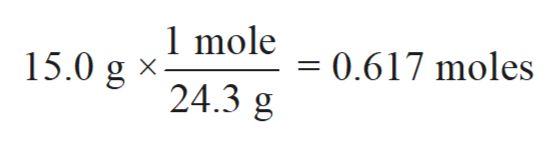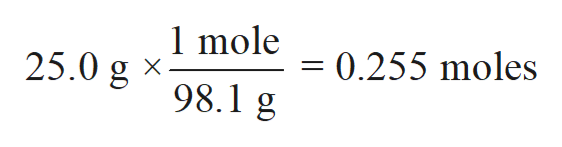Calculate the volume of hydrogen gas at 273 Kelvin and .95atm when 15.0 g of magnesium reacts with 25.0 g of sulfuric acid.

Question

Calculate the volume of hydrogen gas at 273 Kelvin and .95atm when 15.0 g of magnesium reacts with 25.0 g of sulfuric acid.

Step 1

In chemistry, there are many ways to check the deviation of experimental data from the accurate value. These different methods provide the difference between accuracy and precisions of the experimental data which can be further used to calculate the error percentage in the experiment.

Step 2

Calculate moles of magnesium:

Molar mass of Mg= 24.3 g/mol

Mass of Mg = 15.0 ghelp_outlineImage Transcriptionclose1 mole 0.617 moles 15.0 g 24.3 g fullscreen
Step 3

Calculate moles of sulfuric acid:

Molar mass of sulfuric acid...help_outlineImage Transcriptionclose1 mole 25.0 g98.1 g 0.255 moles fullscreen

Want to see the full answer?

See Solution

Want to see this answer and more?

Our solutions are written by experts, many with advanced degrees, and available 24/7

See Solution
Tagged in

Chemistry